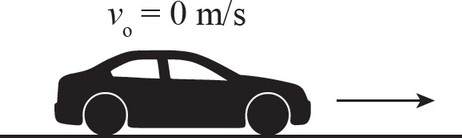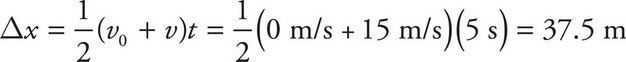# AP Physics 1 Question 425: Answer and Explanation

### Test Information

Question: 4253. A car initially at rest accelerates linearly at a constant rate for 5 s. If the final speed of the car is 15 m/s, what was the displacement of the car?

• A. 20 m
• B. 37.5 m
• C. 75 m
• D. 150 m